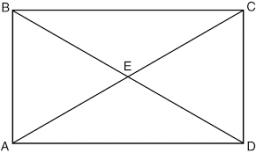# Circumference 5254

Calculate the shorter side and the diagonal of the rectangle if one side is 2 cm longer than the other and its circumference is equal to 70 centimeters.

a =  16.5 cm
u =  24.7891 cm

### Step-by-step explanation:

b=a+2
2(a+b)=70

b=a+2
2·(a+b)=70

a-b = -2
2a+2b = 70

Pivot: Row 1 ↔ Row 2
2a+2b = 70
a-b = -2

Row 2 - 1/2 · Row 1 → Row 2
2a+2b = 70
-2b = -37

b = -37/-2 = 18.5
a = 70-2b/2 = 70-2 · 18.5/2 = 16.5

a = 33/2 = 16.5
b = 37/2 = 18.5

Our linear equations calculator calculates it.Did you find an error or inaccuracy? Feel free to write us. Thank you!

Tips for related online calculators
Do you have a linear equation or system of equations and looking for its solution? Or do you have a quadratic equation?